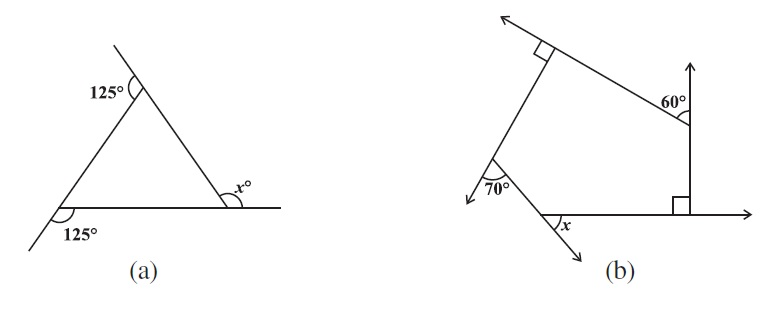# vs.eyeandcontacts.com

## Chapter 3 Understanding Quadrilaterals Exercise 3.2

Question 1: Find x in the following figures.a)
= 125° + 125° + x° = 360° (sum of exterior angles = 360°)
= 250° + x = 360°
= x = 360 - 250
= x = 70°

b)
= 70° + x° + 90° + 60° + 90° = 360° (sum of exterior angles = 360°)
= 310° + x = 360°
= x = 360 - 310
= x = 50°

Question 2: Find the measure of each exterior angle of a regular polygon of
(i) 9 sides
(ii) 15 sides

a)
Number of sides = 9
Measure of exterior angle = ?
Formula = 360°/number of sides
Solution
= 360°/9
= 40°

b)
Number of sides = 15
Measure of exterior angle = ?
Formula = 360°/number of sides
Solution
= 360°/15
= 24°

Question 3: How many sides does a regular polygon have if the measure of an exterior angle is 24°?

Measure of exterior angle = 24°
Number of sides = ?
Formula = 360°/measure of exterior angle = number of sides
Solution
= 360°/24
= 15 sides
Therefore, the regular polygon has 15 sides.

Question 4: How many sides does a regular polygon have if each of its interior angles is 165°?

Measure of interior angle = 165°
Measure of exterior angle = ?
= 180° - 165° = 15° (supplementary angles equal to 180°)
Therefore, the measure of exterior angle is 15°.
Measure of exterior angle = 15°
Number of sides = ?
Formula = 360°/measure of exterior angle = number of sides
Solution
= 360°/15
= 24 sides
Therefore, the regular polygon has 15 sides.

Question 5:
a) Is it possible to have a regular polygon with measure of each exterior angle as 22°?
b) Can it be an interior angle of a regular polygon? Why?

a) The sum of all the exterior angles of a regular polygon is equal to 360° which is not divisible by 22°. Therefore, it is not possible to have a regular polygon with exterior angle as 22°.

b) No, it can be an interior angle of a regular polygon as each exterior angle is 180° - 22° = 158°, which is not a divisor of 360°.

Question 6:
a) What is the minimum interior angle possible for a regular polygon? Why?
b) What is the maximum exterior angle possible for a regular polygon?